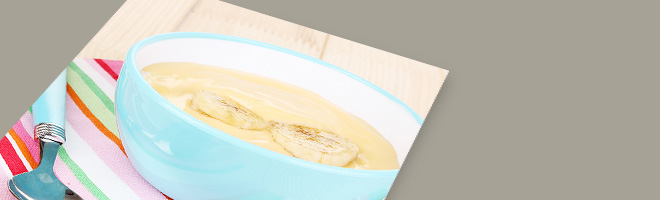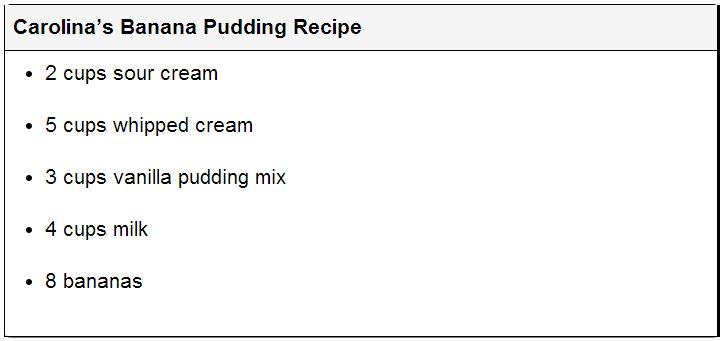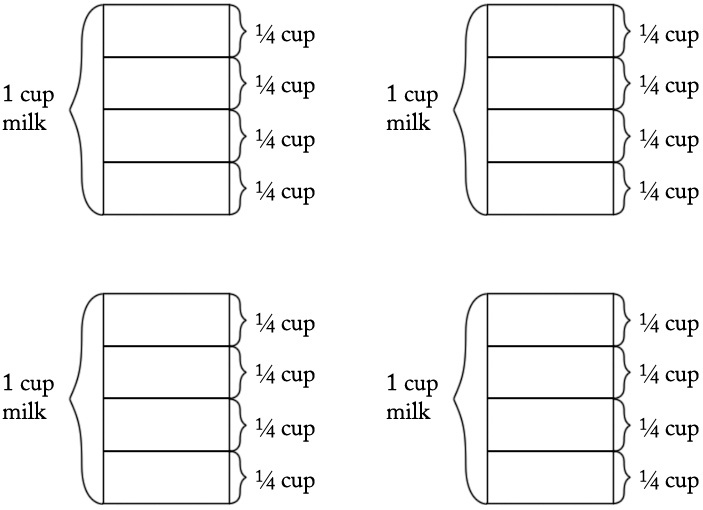• 07/16/14   |   Adjusted: 08/01/18   |   1 file# Banana Pudding

Author: Illustrative Mathematics

• Description
• Files

Mathematically:

• Addresses standards:  5.NF.B.7 and MP.2
• Provides opportunity to use concrete representation to make the mathematics explicit for 5.NF.B.7
• Asks students to connect a visual model to an equation that represents the problem (MP.2)
• Develops the relationship between multiplication and division

In the classroom:

• Prompts students to share their developing thinking and understanding using both illustrations and equations
• Captures student attention by using a real-world context
• Allows for small group, partner, or individual work

This task was designed to include specific features that support access for all students and align to best practice for English Language Learner (ELL) instruction. Go here to learn more about the research behind these supports. This lesson aligns to ELL best practice in the following ways:

• Provides opportunities for students to practice and refine their use of mathematical language.
• Allows for whole class, small group, and paired discussion for the purpose of practicing with mathematical concepts and language.
• Elicits evidence of student thinking both verbally and in written form. • Includes a mathematical routine that reflects best practices to supporting ELLs in accessing mathematical concepts.
• Offers the opportunity for students to act out the problem when the task features complex real-world situations.
• Provides students with support in negotiating written word problems through multiple reads and/or multi-modal interactions with the problem.

• Making the Shifts

How does this task exemplify the instructional Shifts required by CCSSM?

 Focus Belongs to the major work of fifth grade Coherence Builds on key understandings of multiplying a fraction by a whole number (4.NF.B.4); lays foundation for division of fractions by fractions (6.NS.A) Rigor Conceptual Understanding: primary in this task Procedural Skill and Fluency: not targeted in this task Application: primary in this taskCarolina is making her special banana pudding recipe. She is looking for her cup measure, but can only find her quarter cup measure.

1. How many quarter cups does she need for the sour cream? Draw a picture to illustrate your solution, and write an equation that represents the situation.
2. How many quarter cups does she need for the milk? Draw a picture to illustrate your solution, and write an equation that represents the situation.
3. Carolina does not remember in what order she added the ingredients but the last ingredient added required 12 quarter cups. What was the last ingredient Carolina added to the pudding? Draw a picture to illustrate your solution, and write an equation that represents the situation.
• Illustrative Mathematics Commentary and Solution

Commentary:

The purpose of this task is to provide students with a concrete situation they can model by dividing a whole number by a unit fraction. For students who are just beginning to think about the meaning of division by a unit fraction (or students who have never cooked), the teacher can bring in a 1/4 cup measuring cup so that students can act it out. If students can reason through parts (a) and (b) successfully, they will be well-situated to think about part (c) which could yield different solution methods.

Students may need a great deal of practice seeing the connection between the visual representation and the more abstract equations. This task provides an excellent opportunity for teachers to emphasize the relationship between multiplication and division, and if students do not automatically see the connection, the teacher should draw their attention to it.

The approaches described in the solution can be used to make the connection between dividing by a whole number and multiplying by its reciprocal. If students are just beginning to understand the meaning of dividing by a unit fraction, such a conversation should be postponed. However, if they feel comfortable solving these kinds of problems with pictures, this task provides a perfect opportunity to see that dividing a whole number by a unit fraction is the same as multiplying by the reciprocal of the unit fraction. This, in turn, prepares them for the more general result that dividing by any fraction is the same as multiplying by its reciprocal, which students will see in 6th grade.

Solution:

a. Carolina needs 8 quarter cups of sour cream because there are 4 quarter cups in 1 cup, and so it takes $2×4=8$ quarter cups to make 2 cups:This is a "how many groups?" division problem because it asks "How many quarter cups are in 2 cups?"
There are two correct equations:

$2÷\frac{1}{4}=?$

or equivalently:

$?×\frac{1}{4}=2$

We can verify that 8 is the correct solution by noting that $8×\frac{1}{4}=\frac{8}{4}=2$ (4.NF.B.4c)

b. Carolina needs 16 quarter cups of milk because there are 4 quareter cups in 1 cup and so $4×4$ quarter cups in 4 cups, as we see in this picture:Again, this is a "how many groups?" division problem because it asks "How many quarter cups are in 4 cups?" There are two correct equations:

$4÷\frac{1}{4}=?$

or equivalently:

$?×\frac{1}{4}=4$

We can verify that 16 is the correct solution by noting that $16×\frac{1}{4}=\frac{16}{4}=4$

c. We can think of this problem several ways. First, we can ask, "How many cups did I start with if i ended up with 12 quarter cups?" We could write an equation for this:

$?÷\frac{1}{4}=12$

We could also say, "I have 12 quarter cups and I want to know how many cups this is, so I can multiply 12 and $\frac{1}{4}$ to find the number of cups I started with." This can be represented with the following equation:

$12×\frac{1}{4}=?$

Notice that these two equations are equivalent and can both be interpreted in terms of the following picture:Here is yet another approach: If we note that there are 4 quarter cups in 1 cup, we can also think of this as a "how many groups?" division problem. We know that there are a total of 12 quarter cups and that there are 4 quarter cups in a cup (a group), and we want to know how many cups (or how many groups) this makes. Here the equation is:

$12÷4=?$

Since $12÷4=3$, she must have started with the vanilla pudding mix, as that is the only ingredient that requires 3 cups.

This task can also be used to introduce students to division of whole numbers by unit fractions.   It is likely that students will use prior knowledge of multiplication of whole numbers and fractions (4.NF.B.4) to solve these problems. When students use multiplication to solve these problems, it provides an opportunity for the teacher to illustrate the relationship between multiplication and division using a visual model such as a number line to show how two wholes divided into fourths equals eight fourths total. Thus, students are able to see how $\frac{1}{4}×8=2$ is related to $2÷\frac{1}{4}=8$.